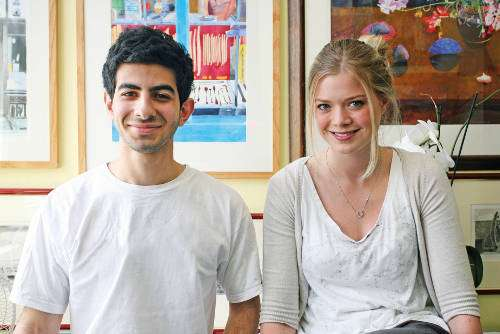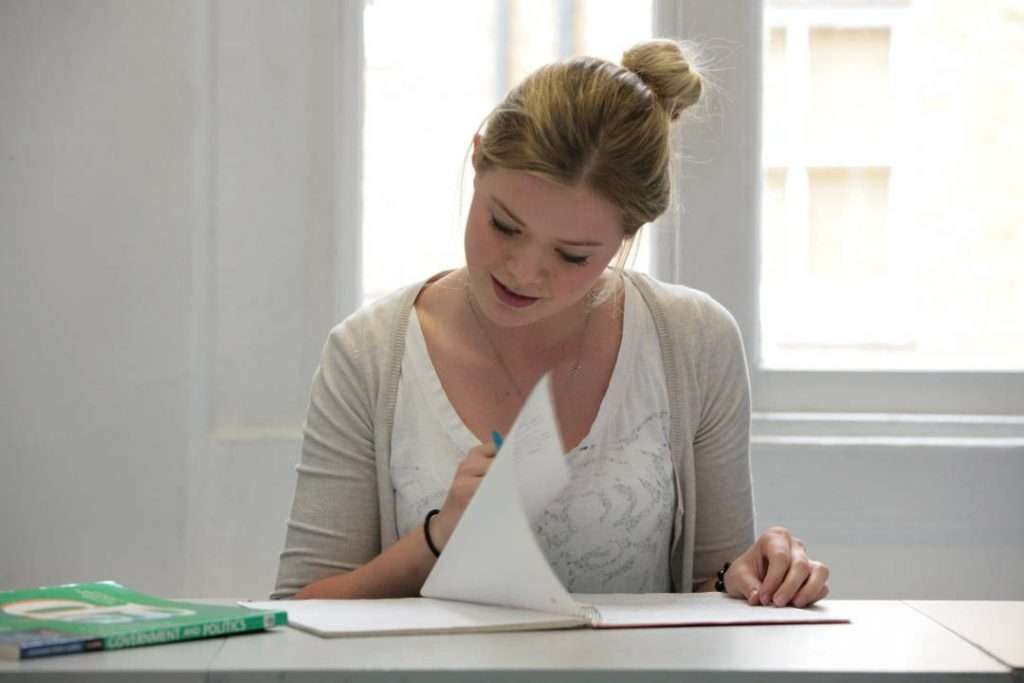# MATHEMATICS AT WESTMINSTER TUTORS

“One of the best sixth forms in London”

## Mathematics – A Level & AS Level Tuition

Mathematics is a very popular subject at Westminster Tutors with a high success rate. Mathematicians seek out patterns to formulate new conjectures, the truth or falsity of which they resolve by mathematical proof. Both pure mathematics, that is mathematics for its own sake, and applied mathematics, such as statistics and mechanics, are taught, and students are able to choose the topics that suit and interest them. Mathematics is crucial in numerous fields, such as natural science, engineering, medicine, finance and the social sciences.

## Course outline

### AS Level Mathematics

Paper 1: Pure Mathematics

• Proof
• Algebra and functions
• Coordinate geometry in the (x, y) plane
• Sequences and series
• Trigonometry
• Exponentials and logarithms
• Differentiation
• Integration
• Vectors

Paper 2: Statistics and Mechanics

Statistics

• Statistical sampling
• Data presentation and interpretation
• Probability
• Statistical distributions
• Statistical hypothesis testing

Mechanics

• Quantities and units in mechanics
• Kinematics
• Forces and Newton’s laws

### A Level Mathematics

Pure Mathematics

• Proof
• Algebra and functions
• Coordinate geometry in the (x, y) plane
• Sequences and series
• Trigonometry
• Exponentials and logarithms
• Differentiation
• Integration
• Numerical methods
• Vectors

Statistics and Mechanics

Statistics

• Statistical sampling
• Data presentation and interpretation
• Probability
• Statistical distributions
• Statistical hypothesis testing

Mechanics

• Quantities and units in mechanics
• Kinematics
• Forces and Newton’s laws
• Moments

### AS Level Further Mathematics

Paper 1: Core Pure Mathematics

• Proof
• Complex numbers
• Matrices
• Further algebra and functions
• Further calculus
• Further vectors

Paper 2: Further Mathematics Options

Further Pure Mathematics 1

• Further trigonometry
• Coordinate systems
• Further vectors
• Numerical methods
• Inequalities

Further Pure Mathematics 2

• Groups
• Further matrix algebra
• Further complex numbers
• Number theory
• Further sequences and series

Further Statistics 1

• Discrete probability distributions
• Poisson & binomial distributions
• Chi squared tests

Further Statistics 2

• Linear regression
• Continuous probability distributions
• Correlation

Further Mechanics 1

• Momentum and impulse
• Work, energy and power
• Elastic collisions in one dimension

Further Mechanics 2

• Motion in a circle
• Centres of mass of plane figures
• Further kinematics

Decision Mathematics 1

• Algorithms and graph theory
• Algorithms on graphs
• Algorithms on graphs II
• Critical path analysis
• Linear programming

Decision Mathematics 2

• Allocation (assignment) problems
• Flows in networks
• Game theory
• Recurrence relations

### A Level Further Mathematics

Core Pure Mathematics

• Proof
• Complex numbers
• Matrices
• Further algebra and functions
• Further calculus
• Further vectors
• Polar coordinates
• Hyperbolic functions
• Differential equations

Further Mathematics Options

Further Pure Mathematics 1

• Further trigonometry
• Further calculus
• Further differential equations
• Coordinate systems
• Further vectors
• Further numerical methods
• Inequalities

Further Pure Mathematics 2

• Groups
• Further calculus
• Further matrix algebra
• Further complex numbers
• Number theory
• Further sequences and series

Further Statistics 1

• Discrete probability distributions
• Poisson & binomial distributions
• Geometric and negative binomial distributions
• Hypothesis testing
• Central Limit Theorem
• Chi Squared Tests
• Probability generating functions
• Quality of tests

Further Statistics 2

• Linear regression
• Continuous probability distributions
• Correlation
• Combination of random variables
• Estimation, confidence intervals and tests using a normal distribution
• Other Hypothesis Tests and confidence intervals
• Confidence intervals and tests using the t – distribution

Further Mechanics 1

• Momentum and impulse
• Work, energy and power
• Elastic strings and springs and elastic energy
• Elastic collisions in one dimension
• Elastic collisions in two dimensions

Further Mechanics 2

• Motion in a circle
• Centres of mass of plane figures
• Further centres of mass
• Further dynamics
• Further kinematics

Decision Mathematics 1

• Algorithms and graph theory
• Algorithms on graphs
• Algorithms on graphs II
• Critical path analysis
• Linear programming

Decision Mathematics 2

• Transportation problems
• Allocation (assignment) problems
• Flows in networks
• Dynamic programming
• Game theory
• Recurrence relations
• Decision analysis

## Assesment

### AS Level Mathematics

Paper 1

• 100 marks
• 2 hours written paper
• 62.5% of total AS level

Paper 2

• 60 marks
• 1 hour 15 minutes written paper
• 37.5% of total AS level

### A Level Mathematics

Paper 1

• Assessment of any topics from the Pure Mathematics content
• 100 marks
• 2 hours written paper
• 33.3% of total A level

Paper 2

• Assessment of any topics from the Pure Mathematics content
• 100 marks
• 2 hours written paper
• 33.3% of total A level

Paper 3

• Assessment of topics from the Statistics and Mechanics content
• 100 marks
• 2 hours written paper
• 33.3% of total A level

### AS Level Further Mathematics

Paper 1

• 80 marks
• 1 hour 40 minutes written paper
• 50% of total AS level

Paper 2

• Assessment of content from two of the options
• 80 marks
• 1 hour 40 minutes written paper
• 50% of total AS level

### A Level Further Mathematics

Paper 1

• Assessment of any topics from the Core Pure Mathematics content
• 75 marks
• 1 hour 30 minutes written paper
• 25% of total A level

Paper 2

• Assessment of any topics from the Core Pure Mathematics content
• 75 marks
• 1 hour 30 minutes written paper
• 25% of total A level

Paper 3

• Assessment of content from one of the Further Mathematics options
• 75 marks
• 1 hour 30 minutes written paper
• 25% of total A level

Paper 4

• Assessment of content from one of the Further Mathematics options
• 75 marks
• 1 hour 30 minutes written paper
• 25% of total A level

## Specification

Although we offer all exam boards as our courses are individually designed, our chosen specification is Edexcel Mathematics (8MA0 and 9MA0) and Further Mathematics (8FM0 and 9FM0). Known for being one of the top colleges in London we believe our results speak for themselves.

## Tutors

Andrew Murray
BSc (Imperial), MA (King’s), MPhil (King’s)

Andrew Niven
BA MEng (Cambridge)

Anthony Korte
MA (Oxford), MSc (UCL), PhD (Imperial)

Helen Wicks
MSc (Imperial), DPhil (Imperial)

Syed Hussain
MSci (Imperial), MSc (Imperial), PhD candidate

Thomas Gregory
MMath (Bath), PhD candidate

Tom Hyatt
MA (Oxford)#### Why Choose Westminster Tutors?

• Top independent sixth form college
• In 2020 A levels 68% A*-A and 92% A*-B
• In 2020 72% placed at Russell Group unis
• Ofsted ‘Outstanding’ across the board
• Superbly qualified teachers who care
• Average A level retake improvement is 2.4 grades#### About Us

Westminster Tutors is one of the oldest and best independent sixth form colleges in London. Established in 1934, our “culture of scholastic excellence” (Ofsted, 2018) is deeply embedded and sets the standard for other colleges to follow.

#### PROSPECTUS

Contact us now to request a prospectus to find out more about Westminster Tutors, all the courses we offer and how we can help you....

#### ADMISSIONS

We are an academically non-selective college and Independent Sixth Form College. We welcome applications from students of all abilities.

#### ABOUT US

Established in 1934, Westminster Tutors is one of the oldest and most respected Independent Sixth Form colleges...

#### LATEST NEWS

College Reopening Date and Exam Replacements

23 Feb, 2021

The government announced yesterday evening that all schools will reopen from Monday 8th March...